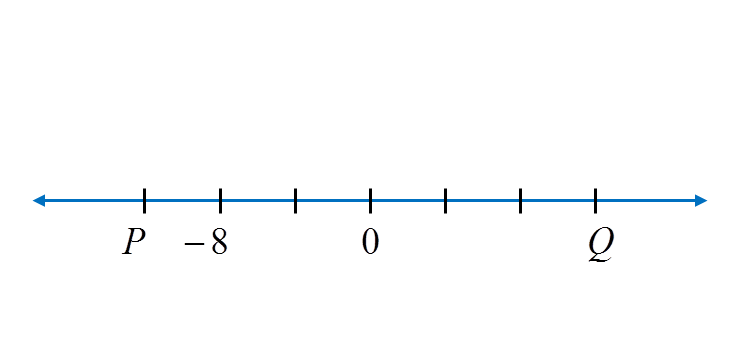# 2.2.1 Number Patterns and Sequences, PT3 Practice

Question 1:
Diagram below is part of a number line.What is the value of P and of Q?

Solution:
Each gradation on the number line represents 4 units. Thus P = –12 and Q = 12.

Question 2:
Diagram below shows a sequence of numbers. K and M represent two numbers.
What are the values of K and M?

Solution:K
= 13 – 6 = 7
M = 1 – 6 = –5

Question 3:
Diagram below shows five integers.Find the sum of the largest integer and the smallest integer.

Solution:
The largest integer = 1
The smallest integer = –10
Sum of the largest integer and the smallest integer
= 1 + (–10)
= –9